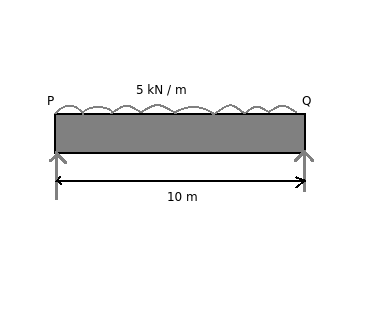# Simply supported beam - Reactions at end points

Q.  Uniformly distributed load of 5 kN acts on a simply supported beam of length 10 m. What are the reactions at end points of the beam?
- Published on 18 Sep 15

a. 12.5 kN
b. 25 kN
c. 50 kN
d. None of the above

#### Discussion

• Sravanthi   -Posted on 14 Dec 15- Uniformly distributed load of 5 kN acts on a simply supported beam of length 10 m as shown above.

Given: Uniformly distributed load = 5 kN, length of beam = 10 m

Solution:

Reactions at point P and Q are same due to symmetrical loading.

Reaction at point P (Rp) = Reactions at point Q (RQ)

Therefore,

(Rp) = (RQ) = (Total downward force acting on beam PQ) / 2

= (5 x 10) / 2

= 25 kN

## ➨ Post your comment / Share knowledgeEnter the code shown above:

(Note: If you cannot read the numbers in the above image, reload the page to generate a new one.)